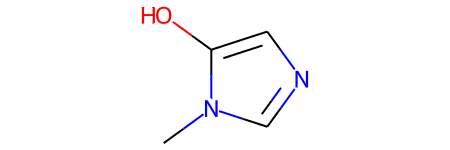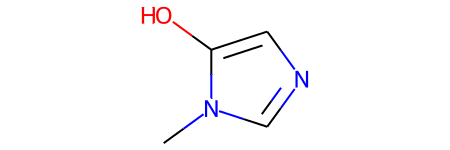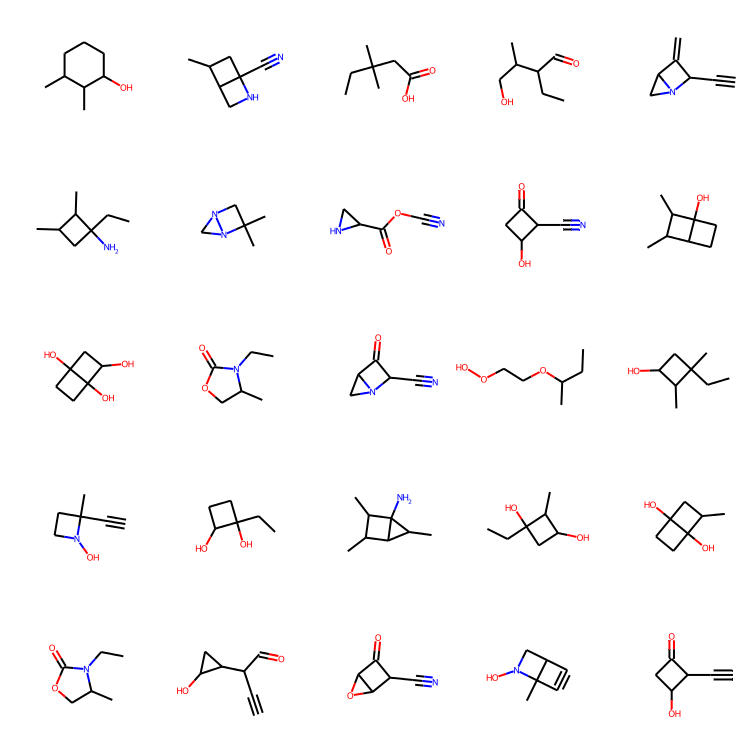Code examples / Generative Deep Learning / WGAN-GP with R-GCN for the generation of small molecular graphs

# WGAN-GP with R-GCN for the generation of small molecular graphs

Author: akensert
Date created: 2021/06/30
Description: Complete implementation of WGAN-GP with R-GCN to generate novel molecules.

ⓘ This example uses Keras 2View in ColabGitHub source

## Introduction

In this tutorial, we implement a generative model for graphs and use it to generate novel molecules.

Motivation: The development of new drugs (molecules) can be extremely time-consuming and costly. The use of deep learning models can alleviate the search for good candidate drugs, by predicting properties of known molecules (e.g., solubility, toxicity, affinity to target protein, etc.). As the number of possible molecules is astronomical, the space in which we search for/explore molecules is just a fraction of the entire space. Therefore, it's arguably desirable to implement generative models that can learn to generate novel molecules (which would otherwise have never been explored).

### References (implementation)

The implementation in this tutorial is based on/inspired by the MolGAN paper and DeepChem's Basic MolGAN.

Recent implementations of generative models for molecular graphs also include Mol-CycleGAN, GraphVAE and JT-VAE. For more information on generative adverserial networks, see GAN, WGAN and WGAN-GP.

## Setup

### Install RDKit

RDKit is a collection of cheminformatics and machine-learning software written in C++ and Python. In this tutorial, RDKit is used to conveniently and efficiently transform SMILES to molecule objects, and then from those obtain sets of atoms and bonds.

SMILES expresses the structure of a given molecule in the form of an ASCII string. The SMILES string is a compact encoding which, for smaller molecules, is relatively human-readable. Encoding molecules as a string both alleviates and facilitates database and/or web searching of a given molecule. RDKit uses algorithms to accurately transform a given SMILES to a molecule object, which can then be used to compute a great number of molecular properties/features.

Notice, RDKit is commonly installed via Conda. However, thanks to rdkit_platform_wheels, rdkit can now (for the sake of this tutorial) be installed easily via pip, as follows:

``````pip -q install rdkit-pypi
``````

And to allow easy visualization of a molecule objects, Pillow needs to be installed:

``````pip -q install Pillow
``````

### Import packages

``````from rdkit import Chem, RDLogger
from rdkit.Chem.Draw import IPythonConsole, MolsToGridImage
import numpy as np
import tensorflow as tf
from tensorflow import keras

RDLogger.DisableLog("rdApp.*")
``````

## Dataset

The dataset used in this tutorial is a quantum mechanics dataset (QM9), obtained from MoleculeNet. Although many feature and label columns come with the dataset, we'll only focus on the SMILES column. The QM9 dataset is a good first dataset to work with for generating graphs, as the maximum number of heavy (non-hydrogen) atoms found in a molecule is only nine.

``````csv_path = tf.keras.utils.get_file(
"qm9.csv", "https://deepchemdata.s3-us-west-1.amazonaws.com/datasets/qm9.csv"
)

data = []
with open(csv_path, "r") as f:
data.append(line.split(","))

# Let's look at a molecule of the dataset
smiles = data
print("SMILES:", smiles)
molecule = Chem.MolFromSmiles(smiles)
print("Num heavy atoms:", molecule.GetNumHeavyAtoms())
molecule
``````
``````SMILES: Cn1cncc1O
Num heavy atoms: 7
``````### Define helper functions

These helper functions will help convert SMILES to graphs and graphs to molecule objects.

Representing a molecular graph. Molecules can naturally be expressed as undirected graphs `G = (V, E)`, where `V` is a set of vertices (atoms), and `E` a set of edges (bonds). As for this implementation, each graph (molecule) will be represented as an adjacency tensor `A`, which encodes existence/non-existence of atom-pairs with their one-hot encoded bond types stretching an extra dimension, and a feature tensor `H`, which for each atom, one-hot encodes its atom type. Notice, as hydrogen atoms can be inferred by RDKit, hydrogen atoms are excluded from `A` and `H` for easier modeling.

``````atom_mapping = {
"C": 0,
0: "C",
"N": 1,
1: "N",
"O": 2,
2: "O",
"F": 3,
3: "F",
}

bond_mapping = {
"SINGLE": 0,
0: Chem.BondType.SINGLE,
"DOUBLE": 1,
1: Chem.BondType.DOUBLE,
"TRIPLE": 2,
2: Chem.BondType.TRIPLE,
"AROMATIC": 3,
3: Chem.BondType.AROMATIC,
}

NUM_ATOMS = 9  # Maximum number of atoms
ATOM_DIM = 4 + 1  # Number of atom types
BOND_DIM = 4 + 1  # Number of bond types
LATENT_DIM = 64  # Size of the latent space

def smiles_to_graph(smiles):
# Converts SMILES to molecule object
molecule = Chem.MolFromSmiles(smiles)

# Initialize adjacency and feature tensor
adjacency = np.zeros((BOND_DIM, NUM_ATOMS, NUM_ATOMS), "float32")
features = np.zeros((NUM_ATOMS, ATOM_DIM), "float32")

# loop over each atom in molecule
for atom in molecule.GetAtoms():
i = atom.GetIdx()
atom_type = atom_mapping[atom.GetSymbol()]
features[i] = np.eye(ATOM_DIM)[atom_type]
# loop over one-hop neighbors
for neighbor in atom.GetNeighbors():
j = neighbor.GetIdx()
bond = molecule.GetBondBetweenAtoms(i, j)
bond_type_idx = bond_mapping[bond.GetBondType().name]
adjacency[bond_type_idx, [i, j], [j, i]] = 1

# Where no bond, add 1 to last channel (indicating "non-bond")
# Notice: channels-first

# Where no atom, add 1 to last column (indicating "non-atom")
features[np.where(np.sum(features, axis=1) == 0), -1] = 1

def graph_to_molecule(graph):
# Unpack graph

# RWMol is a molecule object intended to be edited
molecule = Chem.RWMol()

# Remove "no atoms" & atoms with no bonds
keep_idx = np.where(
(np.argmax(features, axis=1) != ATOM_DIM - 1)
& (np.sum(adjacency[:-1], axis=(0, 1)) != 0)
)
features = features[keep_idx]

for atom_type_idx in np.argmax(features, axis=1):
atom = Chem.Atom(atom_mapping[atom_type_idx])

# Add bonds between atoms in molecule; based on the upper triangles
# of the [symmetric] adjacency tensor
(bonds_ij, atoms_i, atoms_j) = np.where(np.triu(adjacency) == 1)
for (bond_ij, atom_i, atom_j) in zip(bonds_ij, atoms_i, atoms_j):
if atom_i == atom_j or bond_ij == BOND_DIM - 1:
continue
bond_type = bond_mapping[bond_ij]

# https://www.rdkit.org/docs/RDKit_Book.html#molecular-sanitization
flag = Chem.SanitizeMol(molecule, catchErrors=True)
# Let's be strict. If sanitization fails, return None
if flag != Chem.SanitizeFlags.SANITIZE_NONE:
return None

return molecule

# Test helper functions
graph_to_molecule(smiles_to_graph(smiles))
``````### Generate training set

To save training time, we'll only use a tenth of the QM9 dataset.

``````adjacency_tensor, feature_tensor = [], []
for smiles in data[::10]:
feature_tensor.append(features)

feature_tensor = np.array(feature_tensor)

print("feature_tensor.shape =", feature_tensor.shape)
``````
``````adjacency_tensor.shape = (13389, 5, 9, 9)
feature_tensor.shape = (13389, 9, 5)
``````

## Model

The idea is to implement a generator network and a discriminator network via WGAN-GP, that will result in a generator network that can generate small novel molecules (small graphs).

The generator network needs to be able to map (for each example in the batch) a vector `z` to a 3-D adjacency tensor (`A`) and 2-D feature tensor (`H`). For this, `z` will first be passed through a fully-connected network, for which the output will be further passed through two separate fully-connected networks. Each of these two fully-connected networks will then output (for each example in the batch) a tanh-activated vector followed by a reshape and softmax to match that of a multi-dimensional adjacency/feature tensor.

As the discriminator network will recieves as input a graph (`A`, `H`) from either the generator or from the training set, we'll need to implement graph convolutional layers, which allows us to operate on graphs. This means that input to the discriminator network will first pass through graph convolutional layers, then an average-pooling layer, and finally a few fully-connected layers. The final output should be a scalar (for each example in the batch) which indicates the "realness" of the associated input (in this case a "fake" or "real" molecule).

### Graph generator

``````def GraphGenerator(
):
z = keras.layers.Input(shape=(LATENT_DIM,))
# Propagate through one or more densely connected layers
x = z
for units in dense_units:
x = keras.layers.Dense(units, activation="tanh")(x)
x = keras.layers.Dropout(dropout_rate)(x)

# Map outputs of previous layer (x) to [continuous] adjacency tensors (x_adjacency)
# Symmetrify tensors in the last two dimensions

# Map outputs of previous layer (x) to [continuous] feature tensors (x_features)
x_features = keras.layers.Dense(tf.math.reduce_prod(feature_shape))(x)
x_features = keras.layers.Reshape(feature_shape)(x_features)
x_features = keras.layers.Softmax(axis=2)(x_features)

generator = GraphGenerator(
dense_units=[128, 256, 512],
dropout_rate=0.2,
latent_dim=LATENT_DIM,
feature_shape=(NUM_ATOMS, ATOM_DIM),
)
generator.summary()
``````
``````Model: "Generator"
__________________________________________________________________________________________________
Layer (type)                    Output Shape         Param #     Connected to
==================================================================================================
input_1 (InputLayer)            [(None, 64)]         0
__________________________________________________________________________________________________
dense (Dense)                   (None, 128)          8320        input_1
__________________________________________________________________________________________________
dropout (Dropout)               (None, 128)          0           dense
__________________________________________________________________________________________________
dense_1 (Dense)                 (None, 256)          33024       dropout
__________________________________________________________________________________________________
dropout_1 (Dropout)             (None, 256)          0           dense_1
__________________________________________________________________________________________________
dense_2 (Dense)                 (None, 512)          131584      dropout_1
__________________________________________________________________________________________________
dropout_2 (Dropout)             (None, 512)          0           dense_2
__________________________________________________________________________________________________
dense_3 (Dense)                 (None, 405)          207765      dropout_2
__________________________________________________________________________________________________
reshape (Reshape)               (None, 5, 9, 9)      0           dense_3
__________________________________________________________________________________________________
tf.compat.v1.transpose (TFOpLam (None, 5, 9, 9)      0           reshape
__________________________________________________________________________________________________
tf.__operators__.add (TFOpLambd (None, 5, 9, 9)      0           reshape
tf.compat.v1.transpose
__________________________________________________________________________________________________
dense_4 (Dense)                 (None, 45)           23085       dropout_2
__________________________________________________________________________________________________
tf.math.truediv (TFOpLambda)    (None, 5, 9, 9)      0           tf.__operators__.add
__________________________________________________________________________________________________
reshape_1 (Reshape)             (None, 9, 5)         0           dense_4
__________________________________________________________________________________________________
softmax (Softmax)               (None, 5, 9, 9)      0           tf.math.truediv
__________________________________________________________________________________________________
softmax_1 (Softmax)             (None, 9, 5)         0           reshape_1
==================================================================================================
Total params: 403,778
Trainable params: 403,778
Non-trainable params: 0
__________________________________________________________________________________________________
``````

### Graph discriminator

Graph convolutional layer. The relational graph convolutional layers implements non-linearly transformed neighborhood aggregations. We can define these layers as follows:

`H^{l+1} = σ(D^{-1} @ A @ H^{l+1} @ W^{l})`

Where `σ` denotes the non-linear transformation (commonly a ReLU activation), `A` the adjacency tensor, `H^{l}` the feature tensor at the `l:th` layer, `D^{-1}` the inverse diagonal degree tensor of `A`, and `W^{l}` the trainable weight tensor at the `l:th` layer. Specifically, for each bond type (relation), the degree tensor expresses, in the diagonal, the number of bonds attached to each atom. Notice, in this tutorial `D^{-1}` is omitted, for two reasons: (1) it's not obvious how to apply this normalization on the continuous adjacency tensors (generated by the generator), and (2) the performance of the WGAN without normalization seems to work just fine. Furthermore, in contrast to the original paper, no self-loop is defined, as we don't want to train the generator to predict "self-bonding".

``````class RelationalGraphConvLayer(keras.layers.Layer):
def __init__(
self,
units=128,
activation="relu",
use_bias=False,
kernel_initializer="glorot_uniform",
bias_initializer="zeros",
kernel_regularizer=None,
bias_regularizer=None,
**kwargs
):
super().__init__(**kwargs)

self.units = units
self.activation = keras.activations.get(activation)
self.use_bias = use_bias
self.kernel_initializer = keras.initializers.get(kernel_initializer)
self.bias_initializer = keras.initializers.get(bias_initializer)
self.kernel_regularizer = keras.regularizers.get(kernel_regularizer)
self.bias_regularizer = keras.regularizers.get(bias_regularizer)

def build(self, input_shape):
bond_dim = input_shape
atom_dim = input_shape

shape=(bond_dim, atom_dim, self.units),
initializer=self.kernel_initializer,
regularizer=self.kernel_regularizer,
trainable=True,
name="W",
dtype=tf.float32,
)

if self.use_bias:
shape=(bond_dim, 1, self.units),
initializer=self.bias_initializer,
regularizer=self.bias_regularizer,
trainable=True,
name="b",
dtype=tf.float32,
)

self.built = True

def call(self, inputs, training=False):
# Aggregate information from neighbors
x = tf.matmul(adjacency, features[:, None, :, :])
# Apply linear transformation
x = tf.matmul(x, self.kernel)
if self.use_bias:
x += self.bias
# Reduce bond types dim
x_reduced = tf.reduce_sum(x, axis=1)
# Apply non-linear transformation
return self.activation(x_reduced)

def GraphDiscriminator(
):

features = keras.layers.Input(shape=feature_shape)

# Propagate through one or more graph convolutional layers
features_transformed = features
for units in gconv_units:
features_transformed = RelationalGraphConvLayer(units)(
)

# Reduce 2-D representation of molecule to 1-D
x = keras.layers.GlobalAveragePooling1D()(features_transformed)

# Propagate through one or more densely connected layers
for units in dense_units:
x = keras.layers.Dense(units, activation="relu")(x)
x = keras.layers.Dropout(dropout_rate)(x)

# For each molecule, output a single scalar value expressing the
# "realness" of the inputted molecule
x_out = keras.layers.Dense(1, dtype="float32")(x)

discriminator = GraphDiscriminator(
gconv_units=[128, 128, 128, 128],
dense_units=[512, 512],
dropout_rate=0.2,
feature_shape=(NUM_ATOMS, ATOM_DIM),
)
discriminator.summary()
``````
``````Model: "model"
__________________________________________________________________________________________________
Layer (type)                    Output Shape         Param #     Connected to
==================================================================================================
input_2 (InputLayer)            [(None, 5, 9, 9)]    0
__________________________________________________________________________________________________
input_3 (InputLayer)            [(None, 9, 5)]       0
__________________________________________________________________________________________________
relational_graph_conv_layer (Re (None, 9, 128)       3200        input_2
input_3
__________________________________________________________________________________________________
relational_graph_conv_layer_1 ( (None, 9, 128)       81920       input_2
relational_graph_conv_layer
__________________________________________________________________________________________________
relational_graph_conv_layer_2 ( (None, 9, 128)       81920       input_2
relational_graph_conv_layer_1[
__________________________________________________________________________________________________
relational_graph_conv_layer_3 ( (None, 9, 128)       81920       input_2
relational_graph_conv_layer_2[
__________________________________________________________________________________________________
global_average_pooling1d (Globa (None, 128)          0           relational_graph_conv_layer_3[
__________________________________________________________________________________________________
dense_5 (Dense)                 (None, 512)          66048       global_average_pooling1d
__________________________________________________________________________________________________
dropout_3 (Dropout)             (None, 512)          0           dense_5
__________________________________________________________________________________________________
dense_6 (Dense)                 (None, 512)          262656      dropout_3
__________________________________________________________________________________________________
dropout_4 (Dropout)             (None, 512)          0           dense_6
__________________________________________________________________________________________________
dense_7 (Dense)                 (None, 1)            513         dropout_4
==================================================================================================
Total params: 578,177
Trainable params: 578,177
Non-trainable params: 0
__________________________________________________________________________________________________
``````

### WGAN-GP

``````class GraphWGAN(keras.Model):
def __init__(
self,
generator,
discriminator,
discriminator_steps=1,
generator_steps=1,
gp_weight=10,
**kwargs
):
super().__init__(**kwargs)
self.generator = generator
self.discriminator = discriminator
self.discriminator_steps = discriminator_steps
self.generator_steps = generator_steps
self.gp_weight = gp_weight
self.latent_dim = self.generator.input_shape[-1]

def compile(self, optimizer_generator, optimizer_discriminator, **kwargs):
super().compile(**kwargs)
self.optimizer_generator = optimizer_generator
self.optimizer_discriminator = optimizer_discriminator
self.metric_generator = keras.metrics.Mean(name="loss_gen")
self.metric_discriminator = keras.metrics.Mean(name="loss_dis")

def train_step(self, inputs):

if isinstance(inputs, tuple):
inputs = inputs

graph_real = inputs

self.batch_size = tf.shape(inputs)

# Train the discriminator for one or more steps
for _ in range(self.discriminator_steps):
z = tf.random.normal((self.batch_size, self.latent_dim))

graph_generated = self.generator(z, training=True)
loss = self._loss_discriminator(graph_real, graph_generated)

)
self.metric_discriminator.update_state(loss)

# Train the generator for one or more steps
for _ in range(self.generator_steps):
z = tf.random.normal((self.batch_size, self.latent_dim))

graph_generated = self.generator(z, training=True)
loss = self._loss_generator(graph_generated)

)
self.metric_generator.update_state(loss)

return {m.name: m.result() for m in self.metrics}

def _loss_discriminator(self, graph_real, graph_generated):
logits_real = self.discriminator(graph_real, training=True)
logits_generated = self.discriminator(graph_generated, training=True)
loss = tf.reduce_mean(logits_generated) - tf.reduce_mean(logits_real)
return loss + loss_gp * self.gp_weight

def _loss_generator(self, graph_generated):
logits_generated = self.discriminator(graph_generated, training=True)
return -tf.reduce_mean(logits_generated)

# Unpack graphs

# Generate interpolated graphs (adjacency_interp and features_interp)
alpha = tf.random.uniform([self.batch_size])
alpha = tf.reshape(alpha, (self.batch_size, 1, 1, 1))
alpha = tf.reshape(alpha, (self.batch_size, 1, 1))
features_interp = (features_real * alpha) + (1 - alpha) * features_generated

# Compute the logits of interpolated graphs
tape.watch(features_interp)
logits = self.discriminator(
)

# Compute the gradients with respect to the interpolated graphs
return tf.reduce_mean(
)
``````

## Train the model

To save time (if run on a CPU), we'll only train the model for 10 epochs.

``````wgan = GraphWGAN(generator, discriminator, discriminator_steps=1)

wgan.compile(
)

``````
``````Epoch 1/10
837/837 [==============================] - 197s 226ms/step - loss_gen: 2.4626 - loss_dis: -4.3158
Epoch 2/10
837/837 [==============================] - 188s 225ms/step - loss_gen: 1.2832 - loss_dis: -1.3941
Epoch 3/10
837/837 [==============================] - 199s 237ms/step - loss_gen: 0.6742 - loss_dis: -1.2663
Epoch 4/10
837/837 [==============================] - 187s 224ms/step - loss_gen: 0.5090 - loss_dis: -1.6628
Epoch 5/10
837/837 [==============================] - 187s 223ms/step - loss_gen: 0.3686 - loss_dis: -1.4759
Epoch 6/10
837/837 [==============================] - 199s 237ms/step - loss_gen: 0.6925 - loss_dis: -1.5122
Epoch 7/10
837/837 [==============================] - 194s 232ms/step - loss_gen: 0.3966 - loss_dis: -1.5041
Epoch 8/10
837/837 [==============================] - 195s 233ms/step - loss_gen: 0.3595 - loss_dis: -1.6277
Epoch 9/10
837/837 [==============================] - 194s 232ms/step - loss_gen: 0.5862 - loss_dis: -1.7277
Epoch 10/10
837/837 [==============================] - 185s 221ms/step - loss_gen: -0.1642 - loss_dis: -1.5273

<keras.callbacks.History at 0x7ff8daed3a90>
``````

## Sample novel molecules with the generator

``````def sample(generator, batch_size):
z = tf.random.normal((batch_size, LATENT_DIM))
graph = generator.predict(z)
# obtain one-hot encoded adjacency tensor
# Remove potential self-loops from adjacency
# obtain one-hot encoded feature tensor
features = tf.argmax(graph, axis=2)
features = tf.one_hot(features, depth=ATOM_DIM, axis=2)
return [
for i in range(batch_size)
]

molecules = sample(wgan.generator, batch_size=48)

MolsToGridImage(
[m for m in molecules if m is not None][:25], molsPerRow=5, subImgSize=(150, 150)
)
``````## Concluding thoughts

Inspecting the results. Ten epochs of training seemed enough to generate some decent looking molecules! Notice, in contrast to the MolGAN paper, the uniqueness of the generated molecules in this tutorial seems really high, which is great!

What we've learned, and prospects. In this tutorial, a generative model for molecular graphs was successfully implemented, which allowed us to generate novel molecules. In the future, it would be interesting to implement generative models that can modify existing molecules (for instance, to optimize solubility or protein-binding of an existing molecule). For that however, a reconstruction loss would likely be needed, which is tricky to implement as there's no easy and obvious way to compute similarity between two molecular graphs.

Example available on HuggingFace